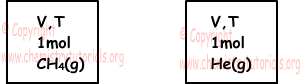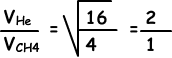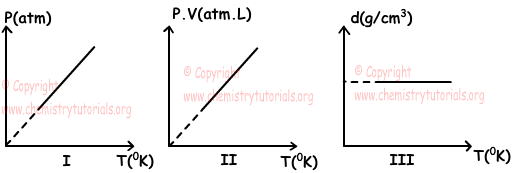## Gases Exam4 and Problem Solutions

Gases Exam4 and  Problem Solutions

1. Find density of C4H8 under 273 0C temperature and 2 atm pressure. (H=1, C=12)

Solution:

T=273 + 273 = 546 0K

P=2atm and Molar mass of C4H8=4.(12) + 8(1)=56g/mol

We use ideal gas law to find density of gas;

P.MC4H8=d.R.T

2.56=d.0,082.546

d=2,5g/L

2. If sum of pressures of 1,6g He and 0,8g CH4 gases is 0,9atm,  find partial pressures of He and CH4.(He=4, C=12, H=1)

Solution:

Moles of gases;

nHe=1,6/4=0,4mol

nCH4=0,8/16=0,05mol

ntotal=0,4 + 0,05=0,45mol

Partial pressures of gases are found by formula; Px=(nx/ntotal).Ptotal

PHe=0,4/0,45,0,0=0,8atm

PCH4=0,05/0,45.0,4=0,1atm

3. There are He gas in given container. Which one of the following statements are true for this container under constant temperature?I. If we add CH4 gas into container, partial pressure of He increases.

II. If we add O2(g) into container, density of the gases in container increases.

III. If we add Ar gas into container, average kinetic energy stays constant.

Solution:

I. Adding CH4 into container increases total number of moles but mole of He does not change, so its partial pressure stays constant. I is false.

II. If we add O2(g) into container, total mass of gases increases and since volume is constant, density of gases increases. II is true.

III. All gases have same average kinetic energy under same temperature. III is true.

4. Which ones of the following statements are true for CH4 and He given in picture below;I. Pressures of CH4 and He are equal.

II. Number of collisions to unit surface in unit time are equal to each other.

III. Their densities are equal.

Solution:

I. Since CH4 and He has equal volume, temperature and mole their pressures are also equal. I is true.

II. Number of collisions in unit time id directly proportional to speed of gases.Speed of He is larger than speed of CH4, so number of collisions of He is larger than number of collisions of CH4.II is false.

III. Since molar masses of CH4 and He are different, their densities are also different. III is false.

5. There are some ideal gas in a closed container having constant volume. Which ones of the graphs given below are true for this gas? (P:Pressure, T:Temperature, d:Density)Solution:

I. Since number of moles and volume of gas are constant, increasing temperature increases pressure. I is true.

II. P.V is directly proportional to temperature and number of moles. Since mole of gas is constant and T increases; P.V also increases. II is true.

III. Since number of moles, volume and mass of gas are constant; density stays constant. III is true.

Related